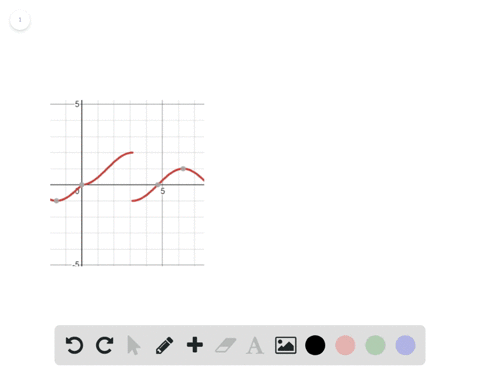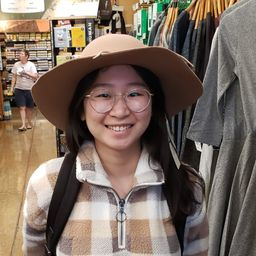🎉 The Study-to-Win Winning Ticket number has been announced! Go to your Tickets dashboard to see if you won! 🎉View Winning Ticket### In Exercises 31 and $32,$ sketch the graph of $f$…

01:28University of California, Berkeley
Problem 31

# In Exercises 31 and $32,$ sketch the graph of $f$ . Then identify the values of $c$ for which $$\lim _{x \rightarrow e} f(x)$$$$f(x)=\left\{\begin{array}{ll}{x^{2},} & {x \leq 2} \\ {8-2 x,} & {2 < x < 4} \\ {4,} & {x \geq 4}\end{array}\right.$$

## Discussion

You must be signed in to discuss.

## Video Transcript

Okay, so it's practical function. See, that with X is less than or equal to our function is X squared. So we know X squared has ordinates. What value did it at two squares? This one I can and then it goes off. Okay. And the next we have eight minutes to act that has an accent is up to ask. For what? We're not gonna include that point. Since we're not included, it's gonna look something like this downward line and made another at X rated very quick before we just have four. So we have a doctor here. This is including four. And I was just going off like that. Okay, so then what value of C makes it so our limits LTD. This is so when the limit as explosions sea of our function. And this is one. Let's see, So every point exists is, except for when we're approaching four. Cracked. Whose only approach from the left hand side. We're looking, though, doesn't the right hand side was looking for. This is when she is not equal to four